## Hydraulic Press Brake For Sale

We have been producing press brakes for more than 20 years and have rich experience. At the same time, we have established deep relationships with various global technology and parts partners to ensure the stable operation of the press brakes we sell.

### NC Press Brake

If you have limit budget, the NC press brake will be a good choice for you to finish sheet metal bending.

### CNC Press Brake

CNC press brake has much higher accuracy, efficiency than NC press brake when bending metal plate.

### Robotic Press Brake

Robotic press brake It can efficiently and accurately complete the automatic bending of sheet metal.

# Metal Stamping And Die Design: Bending

Bend definition

Bending refers to the processing method of bending the product into a certain angle and shape by using a mold in the press production.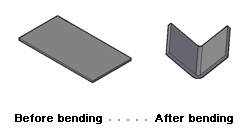Bend example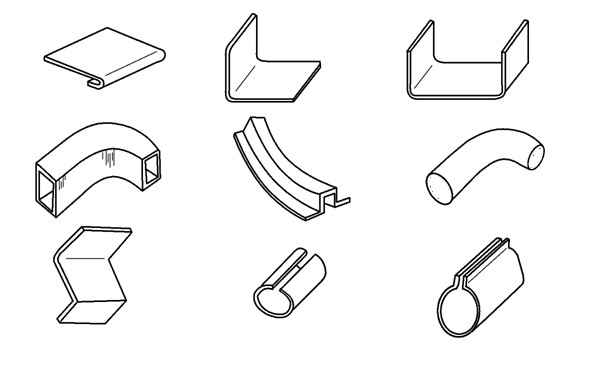Bent parts in life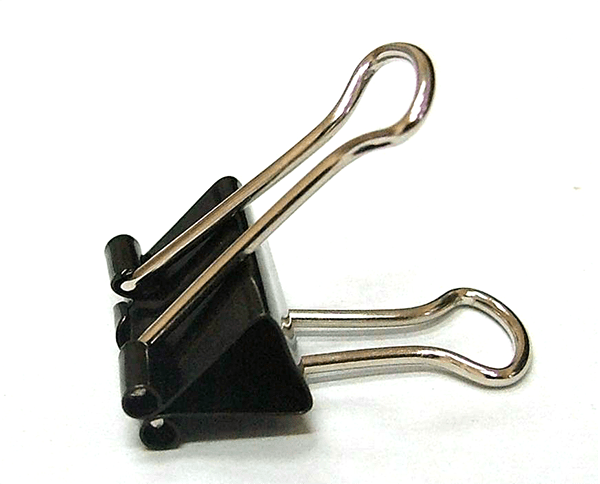Forming curved parts with a mold-1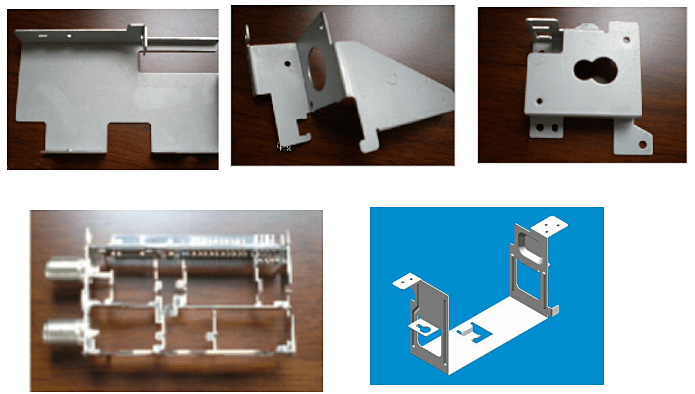Forming bent parts with a mold-2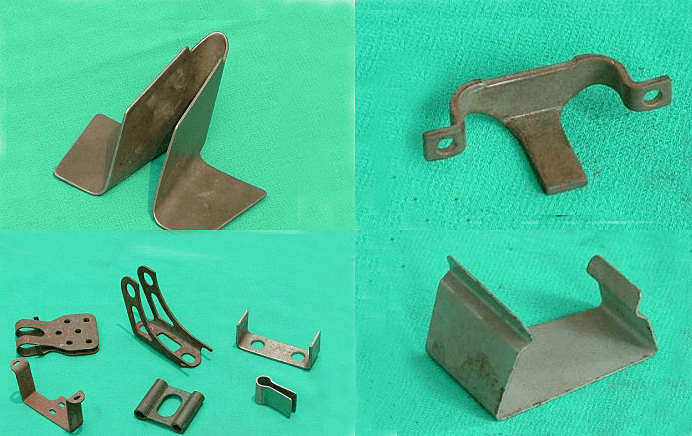The mold used for bending is called bending mold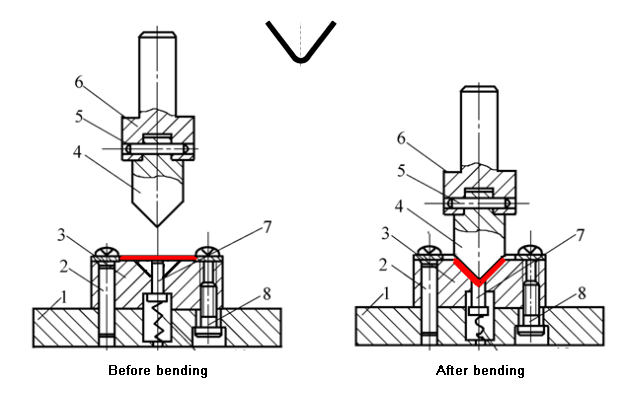## Analysis of bending deformation process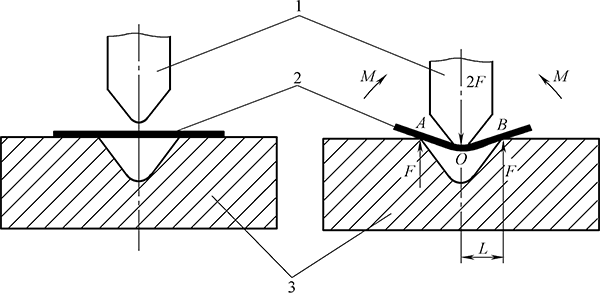Bending process of V-shaped bend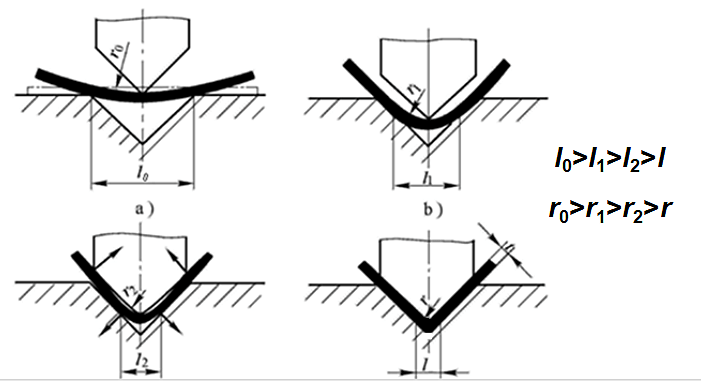### 1.1  Bending way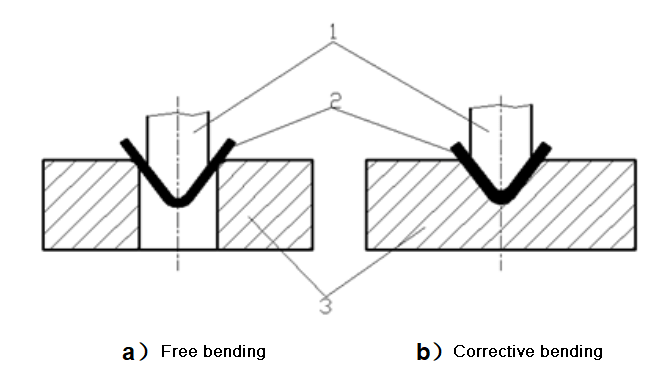### 1.2  Bending deformation characteristics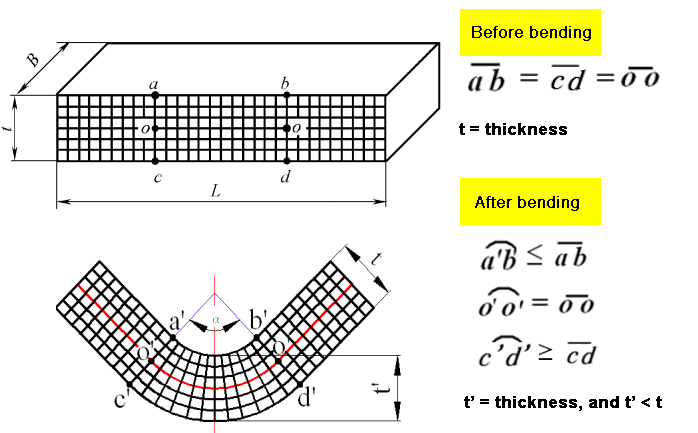Change of cross section of curved blank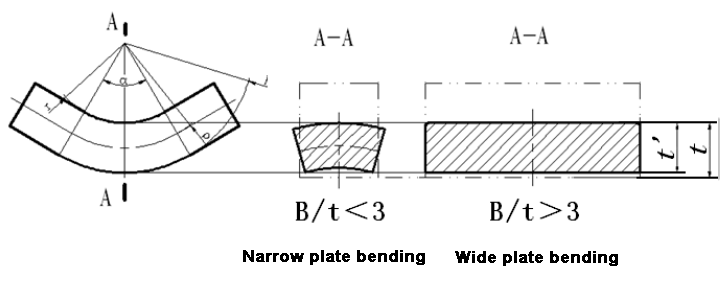Deformation characteristics of bending deformation zone:

• The workpiece is divided into two parts, straight edges and rounded corners. Deformation mainly occurs at the rounded corners. Rounded corners are the main deformation area of bending deformation.
• The deformation zone is not uniformly deformed: the outer zone is stretched in the tangential direction; the inner zone is compressed in the tangential direction, and a strain-neutral layer appears—a metal layer whose length does not change before and after deformation.
• The thickness of the deformation zone becomes thinner, η= t ‘/ t≤1, and the degree of thinning is related to the size of r.
• Changes in cross section: the wide plate remains unchanged, the inner area of the narrow plate becomes wider, and the outer area becomes narrower.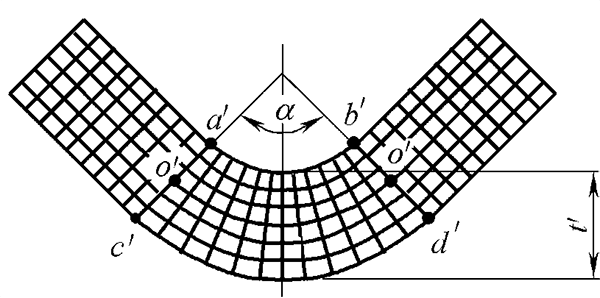### 1.3  State of stress and strain in bending deformation zone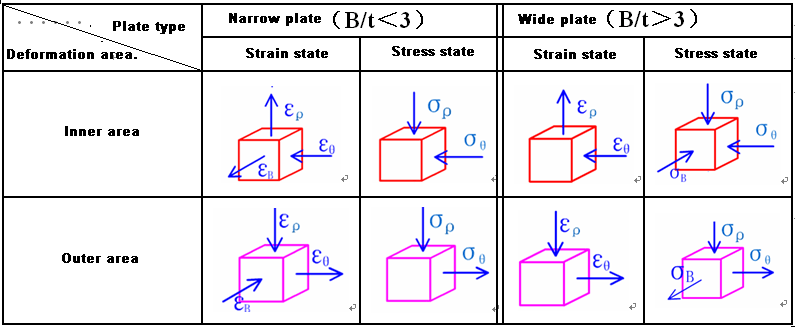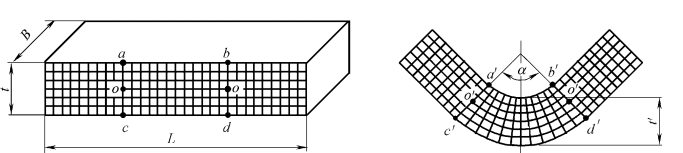## Quality analysis and control of bending parts

### 2.1  Bending crack

Bending crack is the phenomenon of cracks in the outer layer of the material in the bending deformation zone.

The main reason for the occurrence of bending cracks is that the degree of bending deformation exceeds the forming limit of the material being bent.

Cracking can be avoided.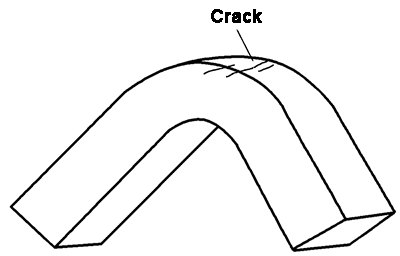1. Bending deformation

r / t——Represents the degree of bending deformation.

The smaller r/t, the greater the degree of bending deformation, there is a minimum relative bending radius rmin /t.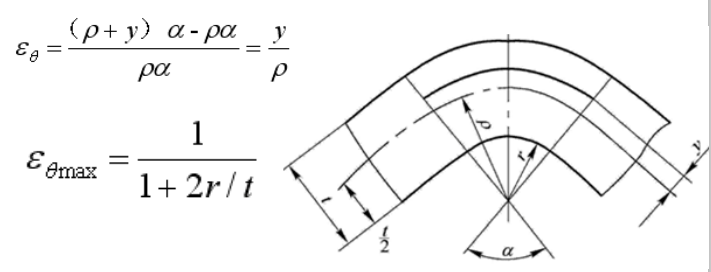1. Minimum relative bending radius and its influencing factors

The minimum relative bending radius refers to the ratio of the bending radius of the outermost fiber to the thickness of the sheet when the sheet is bent and almost crack.

Factors affecting the minimum relative bending radius:

1) Mechanical properties of the material: good plasticity, small rmin/t.

2) The fiber direction of the sheet: the bending line is perpendicular to the fiber direction, rmin/t is small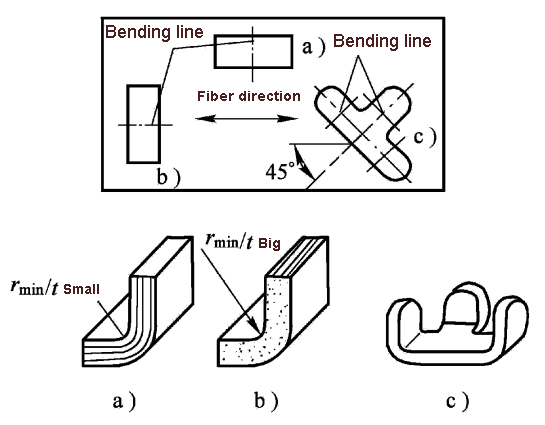3) The surface and side quality of the sheet: the surface and side quality are good, rmin/t is small

4) The thickness of the sheet is thin: rmin/t is small

1. Definition of various parameters of the bending process: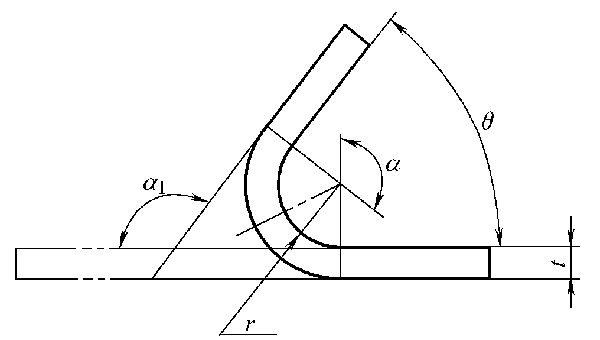(1) The fillet radius r of the bending deformation area is called the bending radius.

(2) The ratio r/t of the bending radius to the sheet thickness is called the relative bending radius.

(3) The bending radius when the outermost fiber of the sheet is near to tearing during bending is called the minimum bending radius rmin.

(4) The ratio of the minimum bending radius to the sheet thickness is called the minimum relative bending radius rmin/t.

(5) The angle at which the workpiece is bent, that is, the complementary angle α1 of the straight angle of the workpiece after bending is called the bending angle.

(6) The diagonal angle α of the straight angle between the bent parts is called the bending center angle.

(7) The angle θ of the straight side of the product after bending is called the angle of the bending part.

1. Measures to control bending

(1) Select a material with good plasticity for bending, and perform an annealing treatment on the cold-work hardened material before bending.

(2) Bending with r/t greater than rmin/t is used.

(3) When arranging, make the bending line perpendicular to the fiber structure direction of the sheet.

(4) Direct the burr side to the side of the bending punch, or remove the burr before bending. Avoid any scratches, cracks and other defects on the outside of the curved blank.

### 2.2  Rebound

Bending rebound refers to the phenomenon that the shape and size of the bent part become inconsistent with the mold when it is taken out of the mold, which is referred to as rebound or springback.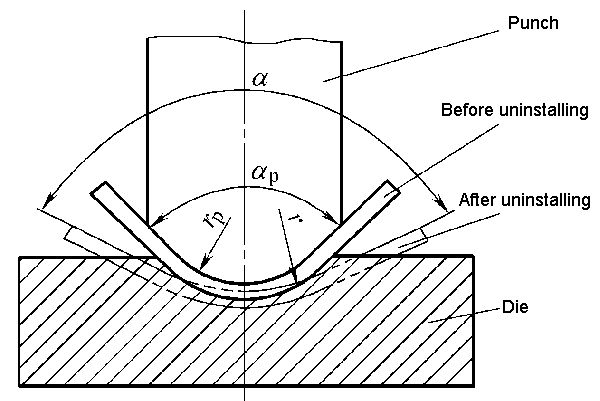The reason for rebound is that the total deformation during plastic bending is composed of two parts: plastic deformation and elastic deformation. When the external load is removed, the plastic deformation remains, and the elastic deformation completely disappears.

1. Rebound form

(2) Change of the angle of the bending piece, the amount of change:

Δα=α-αP

When Δα> 0, it is called positive rebound

When Δα<0, it is called negative rebound

1.  Factors affecting rebound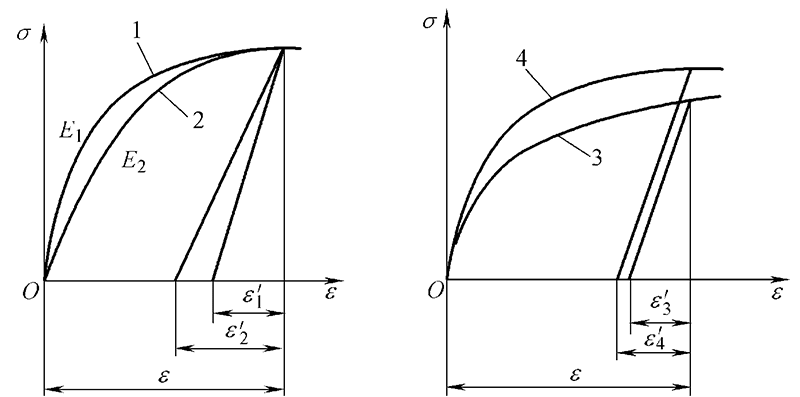1) Mechanical properties of the material: The larger the yield limit and the higher the hardening index, the larger the springback; the larger the elastic modulus, the smaller the springback.

2) The larger the relative bending radius, the greater the rebound.

3) The larger the bending center angle, the longer the length of the deformation zone, and the larger the springback accumulation value, so the springback will increase.

4) Bending method: The springback of correction bending is greatly reduced compared with free bending.

5) Workpiece shape: The more complicated the shape, the more the angle of one bending, the smaller the springback.

6) Mold structure: The springback of the bottom die is small.

1.  Measures to reduce rebound

(1) Improve the design of the bent parts and select the appropriate materials

1) Avoid choosing too large r/t.

2) Try to use a sheet with a small yield limit, a small hardening index, and a large elastic modulus for bending.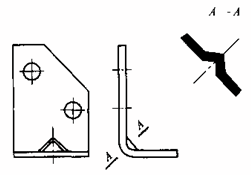(2) Adopt proper bending process to change the stress-strain state of the deformation zone.

1) Use corrective bending instead of free bending.

2) Using the bending process

3) The material for cold work hardening must be annealed first to reduce the yield point σs. For materials with large rebound, heat bending can be used if necessary.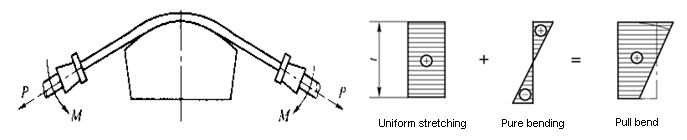(3) Reasonably design the bending die

1) Compensation method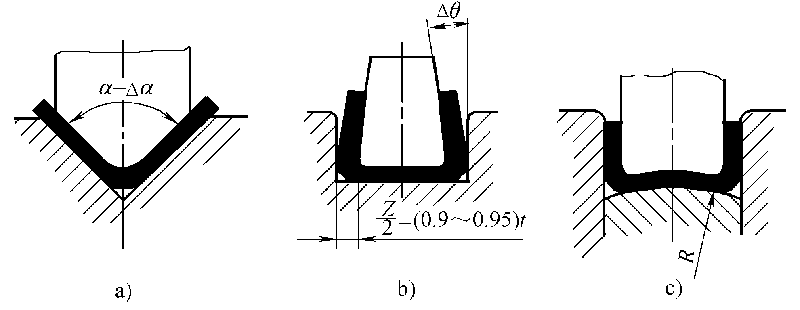2) Make the mold into partial protrusions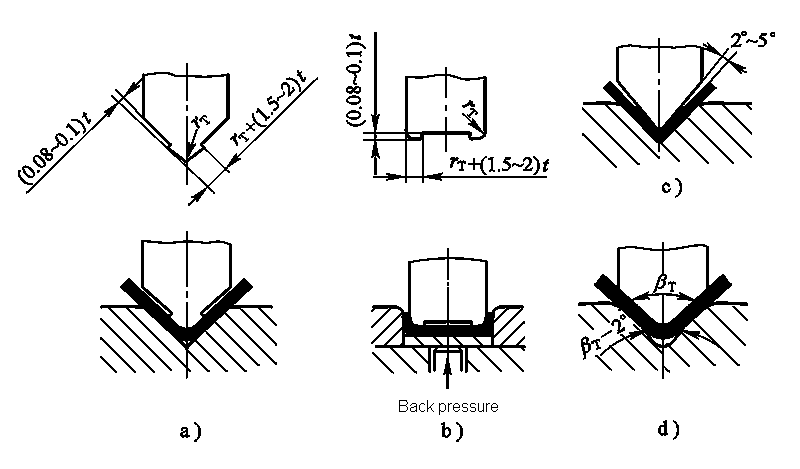3) Soft mold method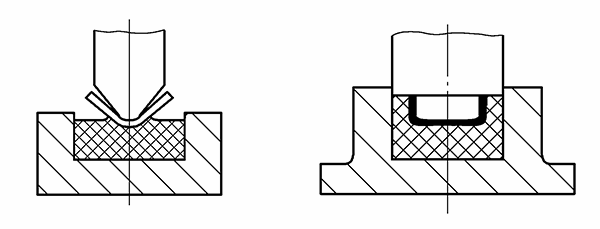### 2.3  Offset

Offset refers to the phenomenon that the sheet blank moves in the mold during the bending process.

As a result of the offset, the length of the two straight sides of the bent part does not meet the requirements of the drawing, so the offset must be eliminated.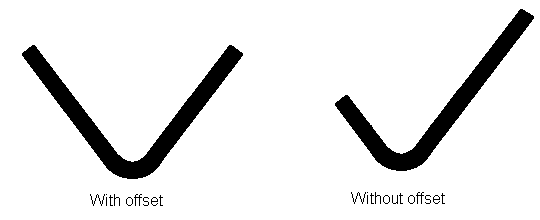1. Reasons for offset

(1) The shape of the blank of the bent part is asymmetrical left and right.

(2) The positioning of the blank is unstable, and the effect of pressing is not ideal.

(3) The mold structure is asymmetrical left and right.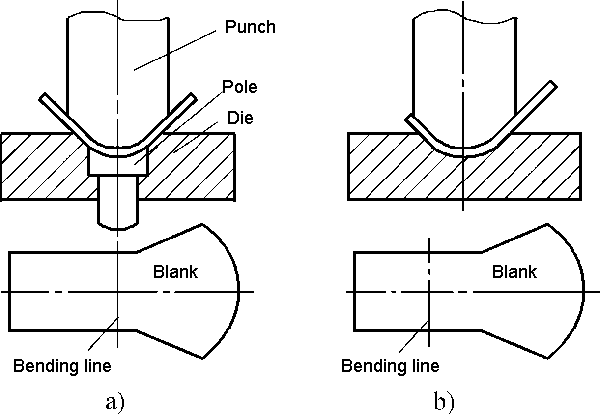1. Measures to control offset

1) Choose a reliable positioning and pressing method, and use a suitable mold structure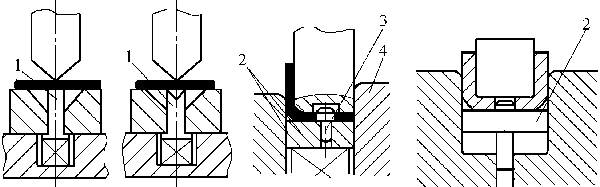2) For small asymmetrical bending parts, the process of paired bending then cutting should be adopted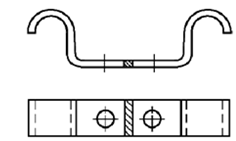### 2.4  Distortion and warpage of sheet cross sections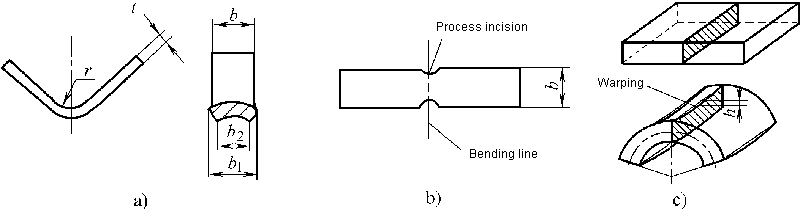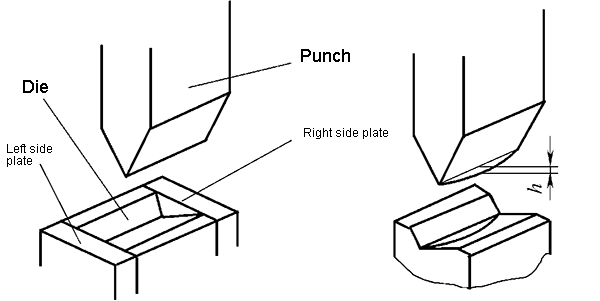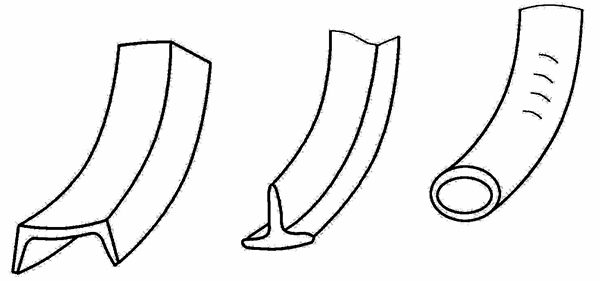### 2.5  Deformation zone becomes thinner and length of bent part increases

This makes it difficult to accurately determine the size of the blank.

The design step of the bending die is to design the bending die first, and then the blanking die.

## Bending process calculation

### 3.1  Calculation of the blank size of the bending part

1. Position of strain neutral layer

The strain-neutral layer refers to a metal layer having a constant length before and after bending deformation or a metal layer with zero tangential strain in a bending deformation region.Equal volume before and after bending: Lbt=π(R2-r2)bα/2π

Simplified: ρ=(r+ηt/2)η

Abbreviated as: ρ=r+χt

2.The length calculation of the blank of the bending part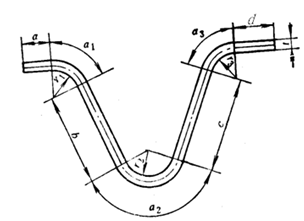(1) Bending pieces with fillet radius r> 0.5t

1) Starting from one end of the bending piece, divide it into several straight and circular segments.

2) Find the neutral layer displacement coefficient χ according to Table 4-3.

3) Determine the bending radius ρ of the neutral layer of each arc segment according to formula (4-3)

4) According to the bending radius ρ1, ρ2 of each neutral layer and the corresponding bending center angles α1, α2 …, calculate the length of each arc segment ll, l2 …  li=πρiαi/180°

5) Calculate the total expansion length L = a + b + c + … + l1 + l2 + l3 + …

(2) Bends with fillet radius r <0.5t—empirical formula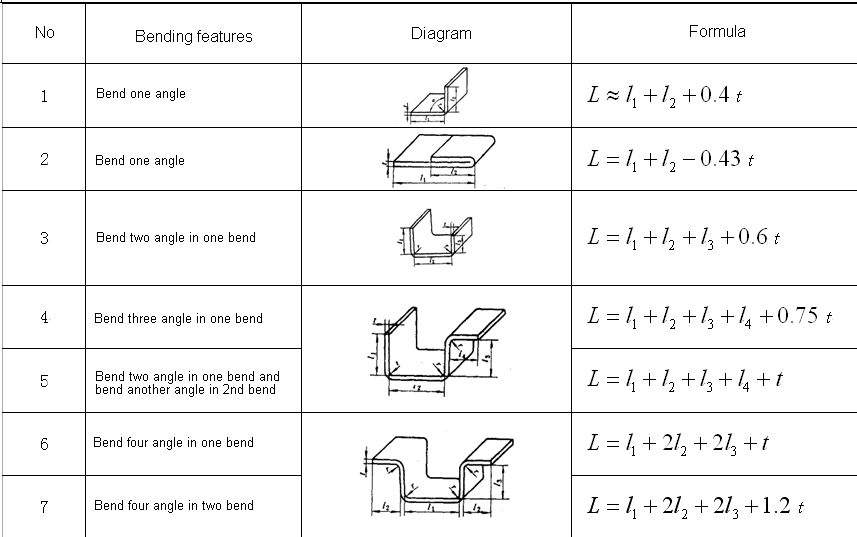Example of calculating the unfolded length of a curved part

Example 4-1 Bend the workpiece shown in Figure 4-30 and try to calculate its unfolded length.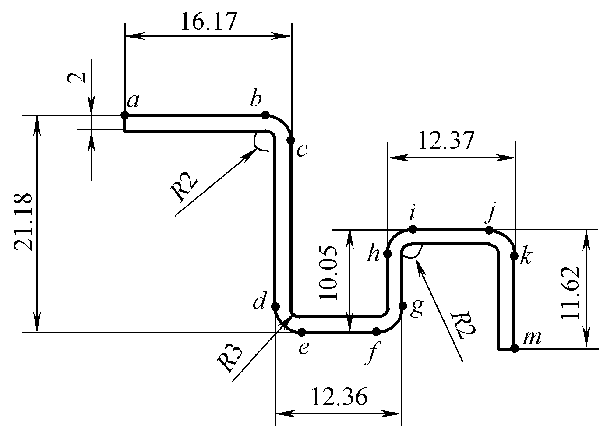Solution: (1) The workpiece is divided into straight line segments lab, lcd, lef, lgh, ljj, lkm and arc segments lbc, lde, lfg, lhi, ljk from point a.

(2) Calculate the extended length of the arc segment.

For the arcs lbc, lhi, ljk: R = 2mm, t = 2mm, then r/t = 2/2 = 1, and if χ= 0.3 is found in Table 4-3, then:

Arc length lbc = lhi = ljk = (2土0.3 × 2) × π/2 = 4.082 (mm)

For the arc lde, lfg: R = 3mm, t = 2mm, then r/t = 3/2 = 1.5. According to Table 4-3, χ= 0.36, then:

Arc length lde = lfg = (2 ± 0.36 × 2) × π/ 2 = 5.84 (mm)

(3) Calculate the total length of the bending blank:

L = ∑/ l straight edge + ∑l rounded corner = lab + lcd + lef + lgh + lij + lkm + lbc + lde + lfg + lhi + ljk

= 16.17-4 + 21.18-9 + 12.36-10 + 10.05-9 + 12.37-8 + 11.62-4 + 3 × 4.802 + 2 × 5.84 = 65.836mm

### 3.2  Calculation of bending process force

1.  Calculation of bending force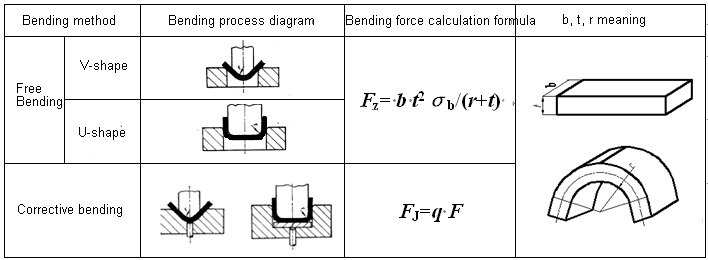Calculation of pressing force or ejecting force

• Pressing force: FY=CYFZ
• Ejecting force: FD=CDFZ
1. Determination of the nominal pressure of the press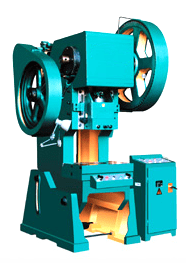For free bending with pressing, the tonnage selection of the press needs to consider the bending force and the pressing force, that is:

Fpress≥1.2(Fz+FY)

For the correction of bending, only the correction of bending force can be considered when selecting the tonnage of the press, that is:

Fpress≥1.2FJ

Selection example of press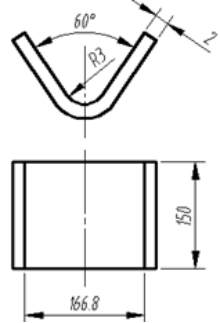Example 4-2 Bend the V-shaped part shown in Figure 4-32. The known material is 20 steel and the tensile strength is 400 MPa. Try to calculate the free bending and correct the bending force respectively. When using the press device, try to select the tonnage of the press.

Solution: From the formula in Table 4-6:

When bending freely: FZ = b * t2σb / (r + t) = 150 × 2 × 2 × 400 / (3 + 2) = 48000 (N)

FY = CYFZ = 0.4 × 48000 = 19200 (N)

Then the total process power is: FZ + FY = 48000 + 19200 = 67.2 (KN) then the equipment tonnage: Fpress ≥ 1.2 (FZ + FY) = 1.2 × 67.2 = 80.64 (KN).

When bending is corrected, q can be taken as 50MPa from Table 4-7, and it can be obtained from the formula in Table 4-6:

FJ = q * A = 50 × 166.8 × 150 = 1251 (KN)

Then the equipment tonnage: Fpress ≥ 1.2 * FJ = 1.2 × 1251 = 1501.2 (KN).

## Bending process design

### 4.1  Bending process analysis

The manufacturability of the bending part refers to whether the shape, size, accuracy, materials, and technical requirements of the bending part meet the technological requirements of the bending process, that is, the adaptability of the bending part to the bending process—a requirement from the perspective of product design.

1. Shape requirements for curved parts

(1) To prevent displacement during bending, it is required that the shape and size of the bending piece are as symmetrical as possible.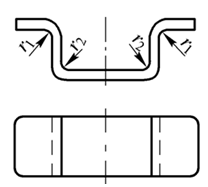(2) When bending a section of edge locally, in order to avoid tearing of the root of the bend, a groove should be cut between the bent part and the unbent part or the process hole should be punched out before bending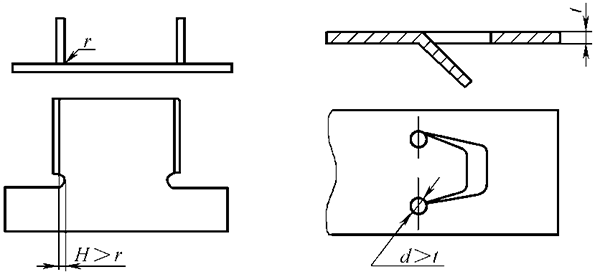(3) Add connection straps and positioning process holes.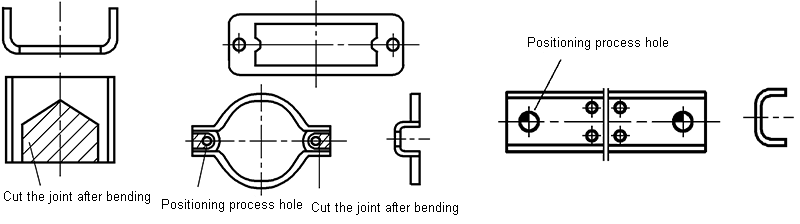2.Dimensional requirements for bent parts

(1) The bending radius should not be smaller than the minimum bending radius.

(2) The height of the straight side of the curved part should meet: h> r + 2t

(3) The distance between the edge of the hole of the bending part should meet the following requirements: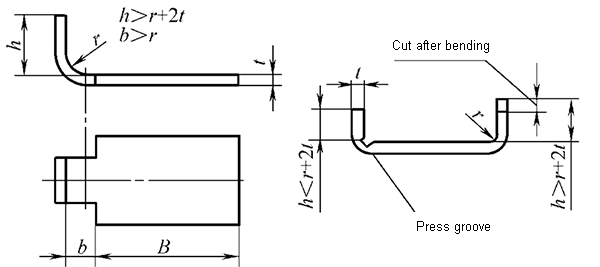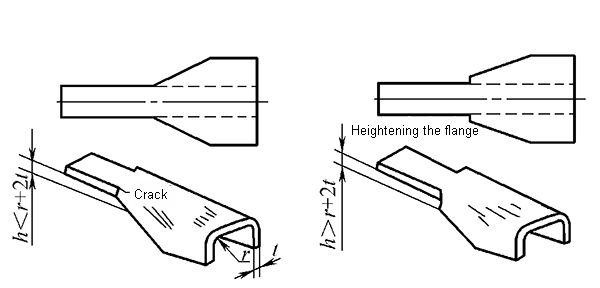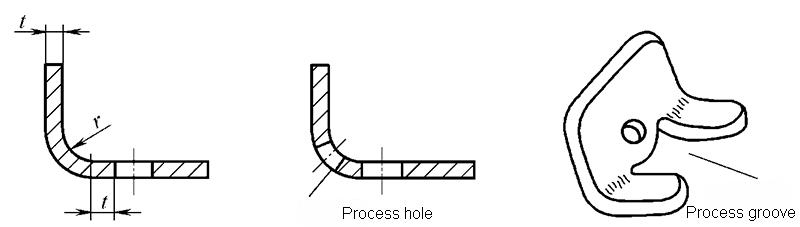1. Precision requirements for bending parts

The dimensional tolerance of the bent parts must conform to GB / T13914-2002,

Angle tolerance accords with GB / T13915-2002,

Unmarked position tolerance conforms to GB / T13916-2002,

The limit deviation of the dimensions without tolerances conforms to GB / T15055-2007

1. Material requirements for curved parts

The material of the bending part must have good plasticity, a small yield ratio, and a large elastic modulus

1. Requirements for dimensioning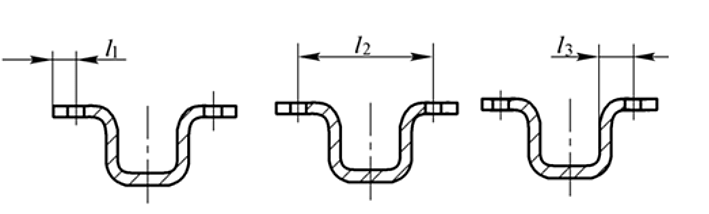### 4.2  Process arrangement of bending parts

1) Simple curved parts: one-time bending. Bending parts with complex shapes: Two or more bending forms.

2) Bending parts with large batch size and small size: Use progressive die or compound die as much as possible.

3) When multiple bends are required: bend both ends first, and then bend the middle part. The previous bend should take into account the reliable positioning of the latter bend.

4) When the shape of the bending piece is not symmetrical: bend as much as possible, and then cut it.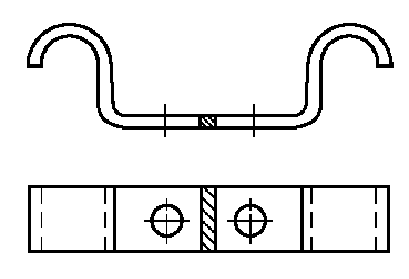Process arrangement of typical bending parts

One bend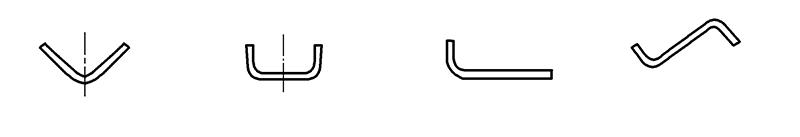Twice bend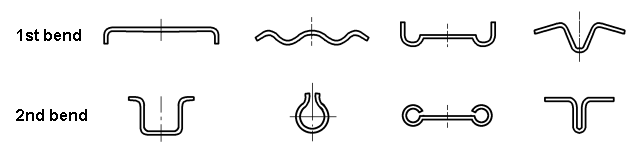Triple bend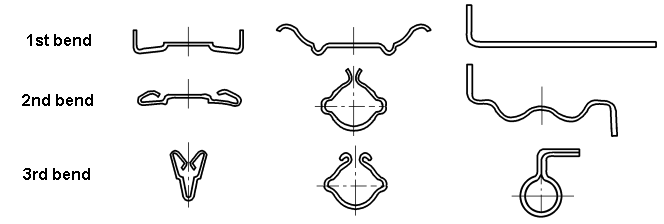Four bends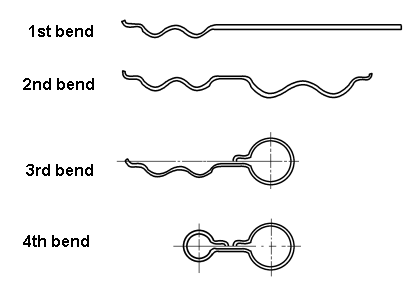Flexible arrangement of bending parts

• Shape of the workpiece
• Precision requirements
• Batch size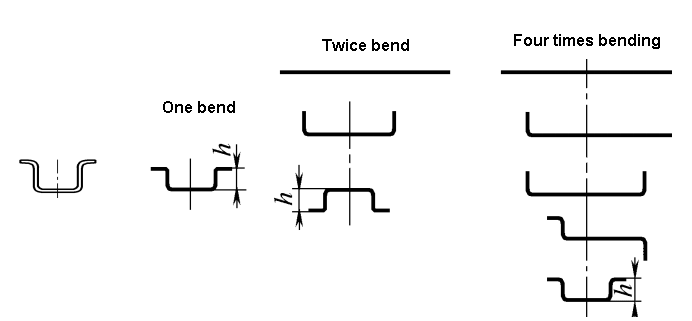## Bending mold design

### 5.1  Bending mold type and structure

According to the degree of process combination, the bending die can be divided into:

• Single process bending die
• Compound bending die
• Progressive bending die

According to the shape of the workpiece, the bending die can be divided into:

• V-shaped bending die
• L-shaped bending die
• U-shaped bending die
• Z-shaped bending die
• Round bending die
• Bending mold for hinge
1. V-shaped bending die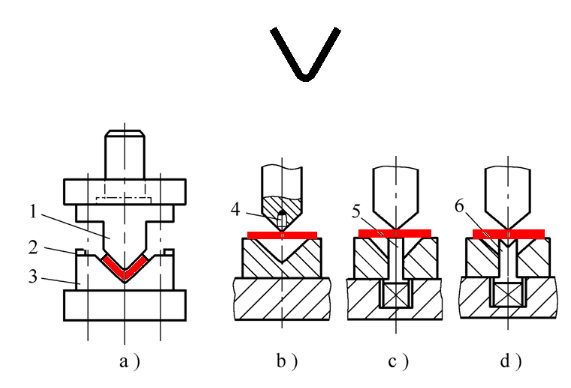V-shaped precision bending die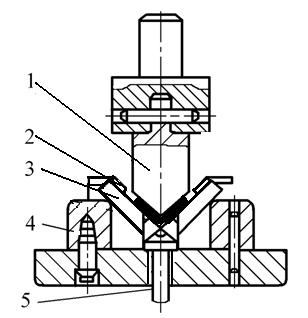• 1- punch
• 2-positioning plate
• 3-moving die
• 4- support plate
• 5- ejector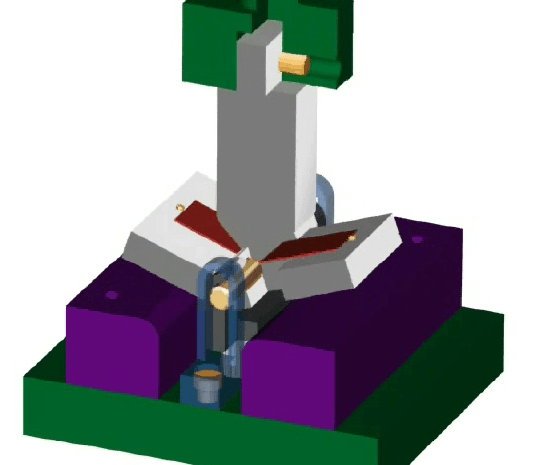V-shaped precision bending die

1. L-shaped bending die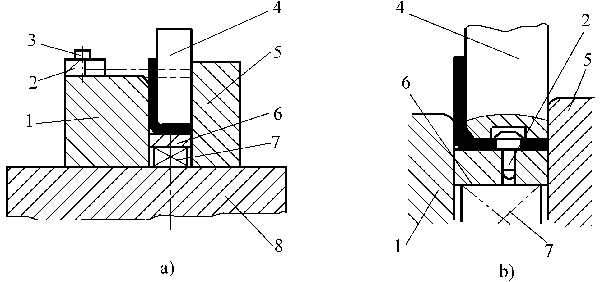3.U-shaped bending die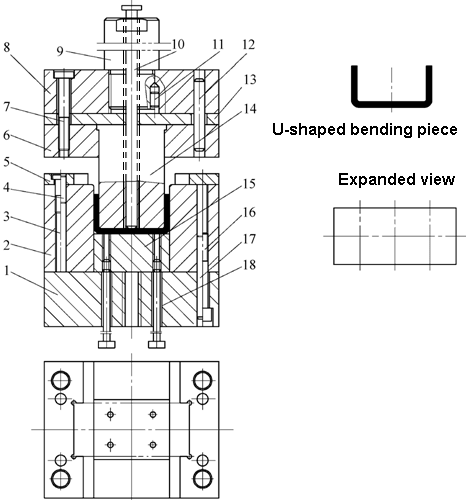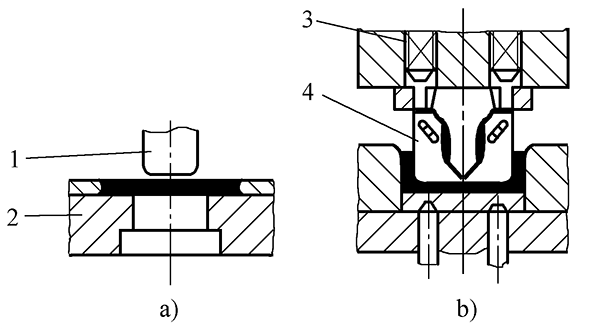Bending die for closed angle pieces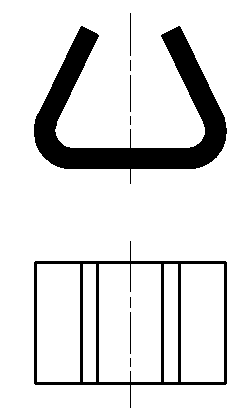Closed angle bending die-1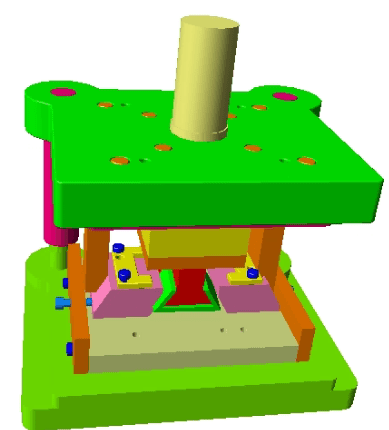Closed angle bending die-2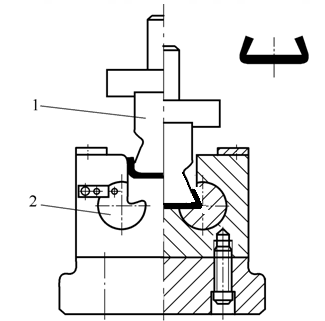1-male die 2-rotating female die 3-spring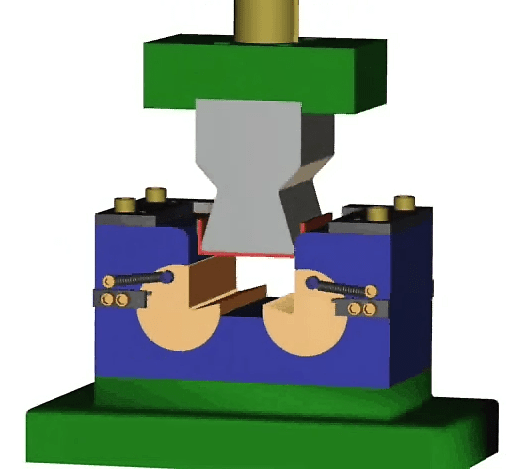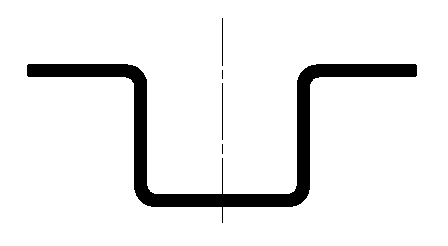Quadrilateral forming bending die at one time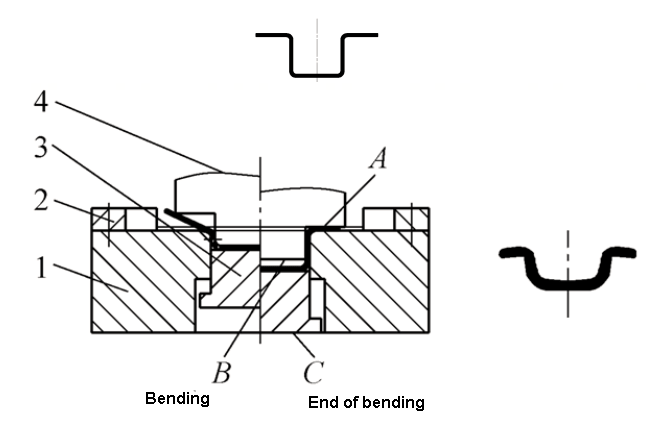Bending die for quadrilateral twice forming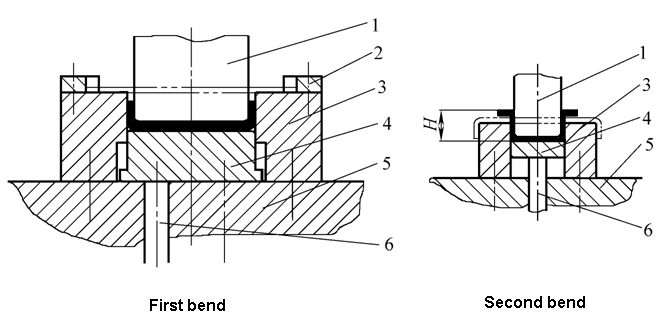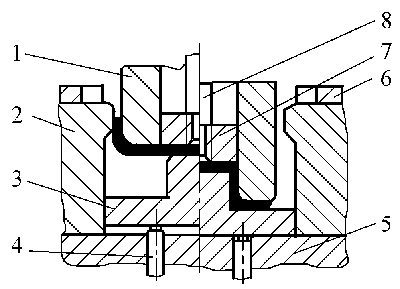• 1-Concave and concave die
• 2-Female die
• 3-Moving punch
• 4-Ejector
• 5-Lower mold base
• 6-Positioning board
• 7-Push block
• 8-Push rod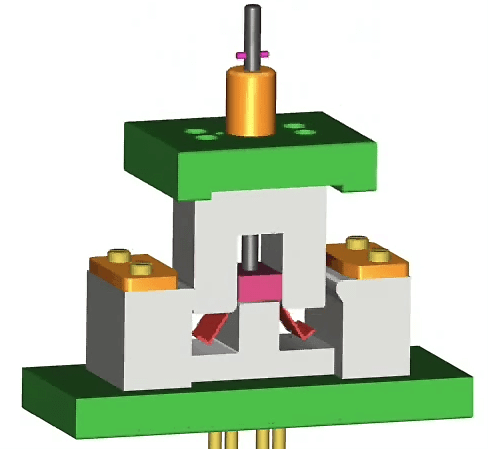• 1-Die
• 2-movable punch
• 3-Pendulum block
• 4-Backing plate
• 5-Pusher block

Bending die of concave die swing quadrilateral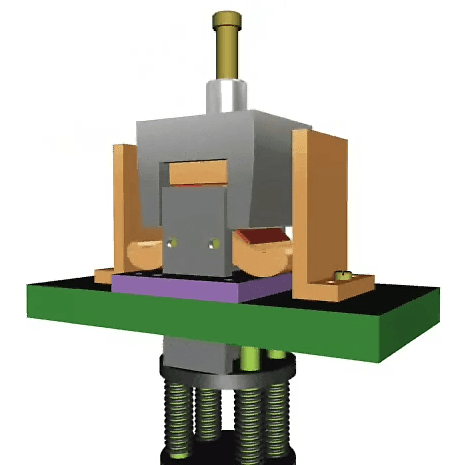1. Z-shapedbending die

Z-shaped one-time bending die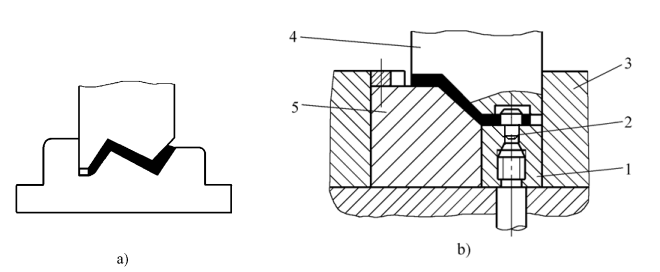Bending die for bending Z-shaped parts in two steps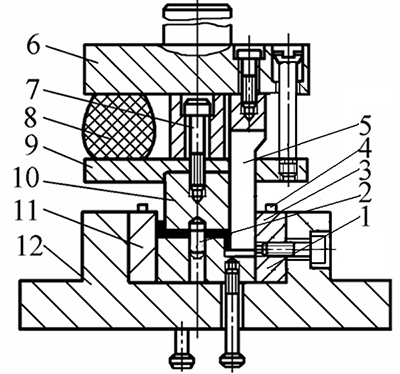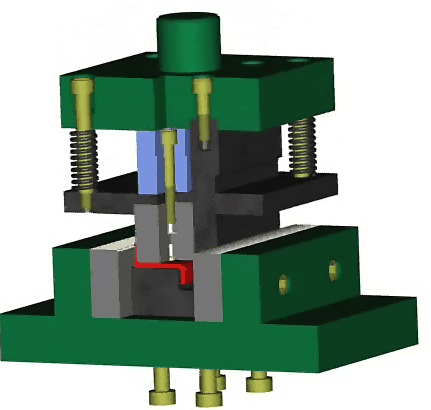1. Round bending die

Round bending die-twice bend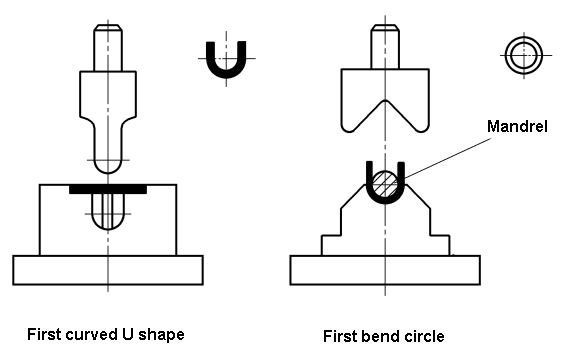Round bending die-one bend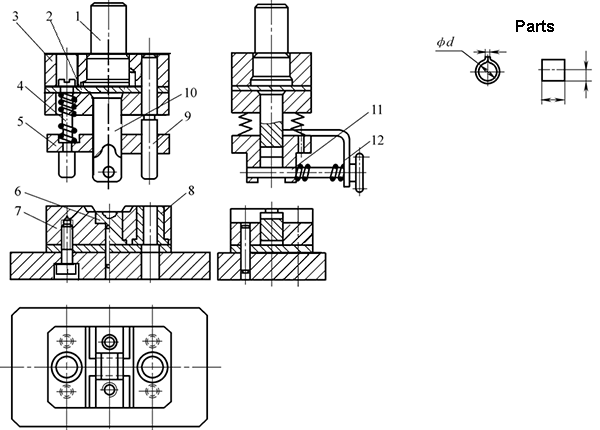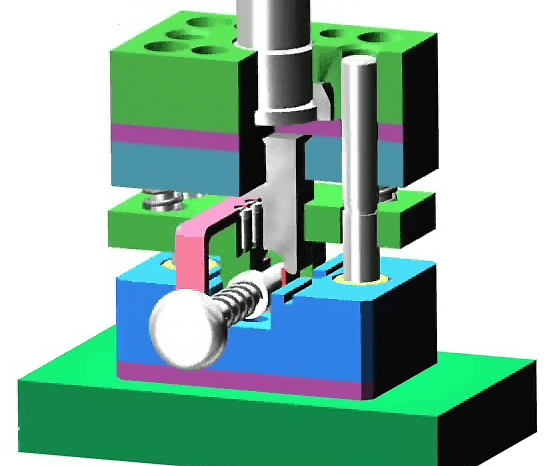One-time bending forming die for circular piece with swing die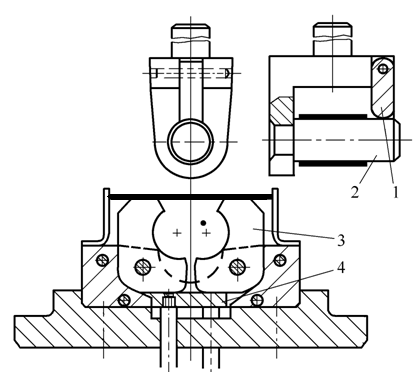• 1- support
• 2-punch
• 3-swing die
• 4-ejector plate

One-time bending forming die for large round pieces with swinging die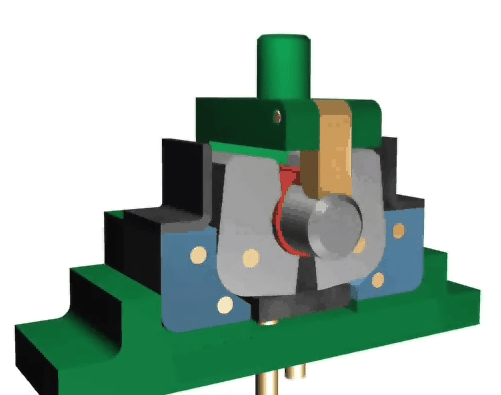Two processes bending big circle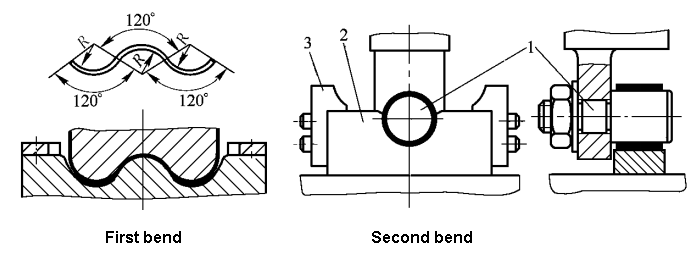Three steps bending big circle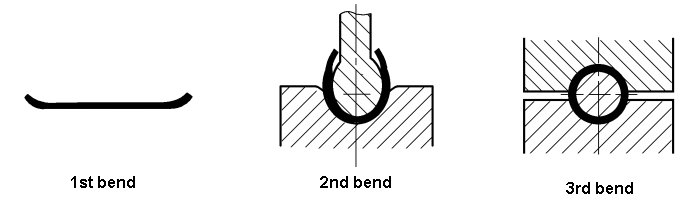1. Bending mold for hinge

Hinge piece two-time bending die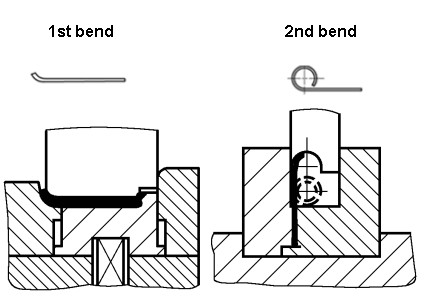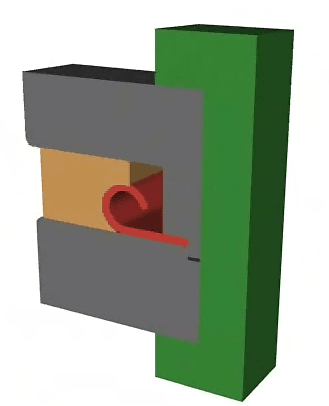Hinge piece one-time bending die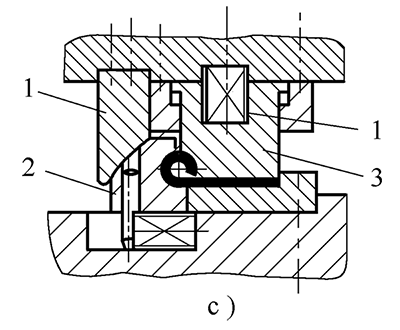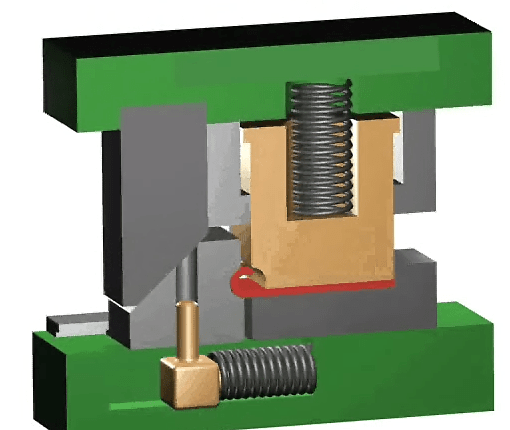1. Other bending dies

(1) Cutting and bending composite mold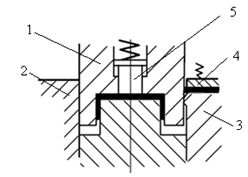(2) Progressive bending die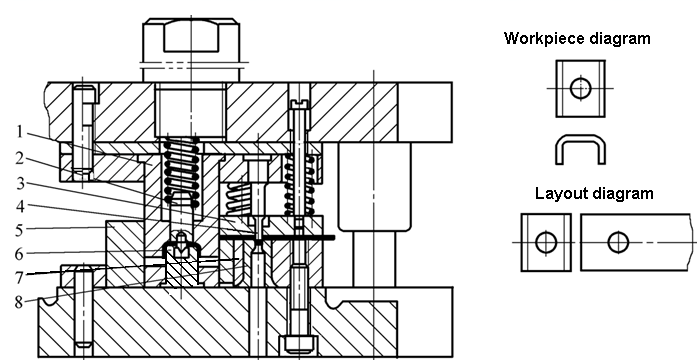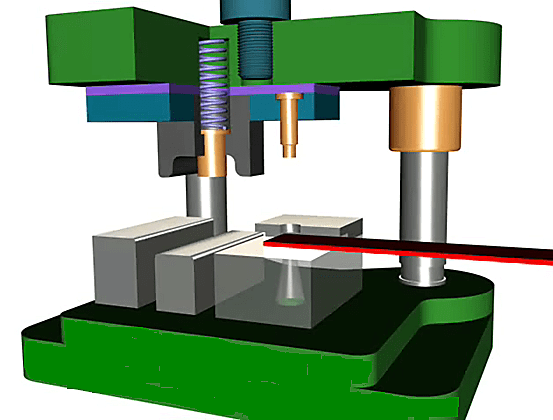### 5.2  Bending mold part design

1. Design of working parts

(3) Die depth

(4) Clearance of convex and concave die

(5) Width of U-shaped convex and concave die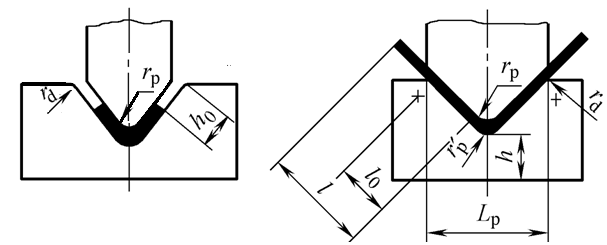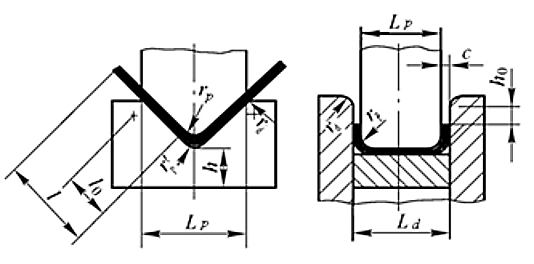1) When r≥rmin, take rp = r, where rmin is the minimum bending radius allowed by the material.

2) When r <rmin, take rp> rmin. The fillet radius r of the workpiece is obtained by shaping, even if the fillet radius rz of the shaping punch is equal to the fillet radius r of the workpiece.

3) When r/t> 10, springback should be considered and the radius of the fillet radius of the punch should be corrected.

4) The bottom of the V-shaped bending die can be opened or retracted with groove or a fillet radius: r’p = (0.6-0.8) (rp + t).

The size of the corner radius of the die affects the bending force, the life of the bending die and the quality of the bending part during the bending process.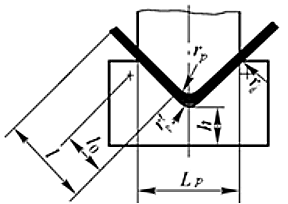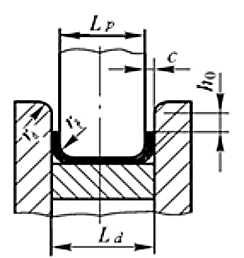• When t≤2mm, rd= (3-6) t
• When t = 2-4mm, rd= (2-3) t
• When t> 4mm, rd= 2t

（3）Die depth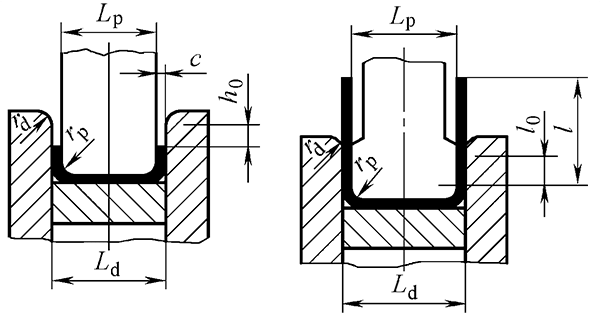（4）Convex and concave die clearance c

The size of the gap between the male and female die affects the bending force, the life of the bending die, and the quality of the bending part.

• Steel plate c = （05 ~ 1.15）t
• Non-ferrous metals c = （1 ~ 1.1）t

When the accuracy of the bending part is high, the gap value should be appropriately reduced, and c = t can be taken.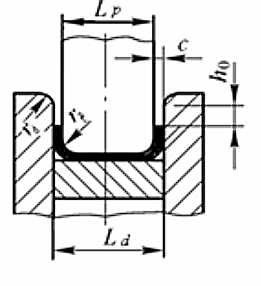The die clearance of the V-shaped bending piece does not need to be designed. It can be obtained by adjusting the closing height of the press.

（5）U-shaped curved convex and concave die width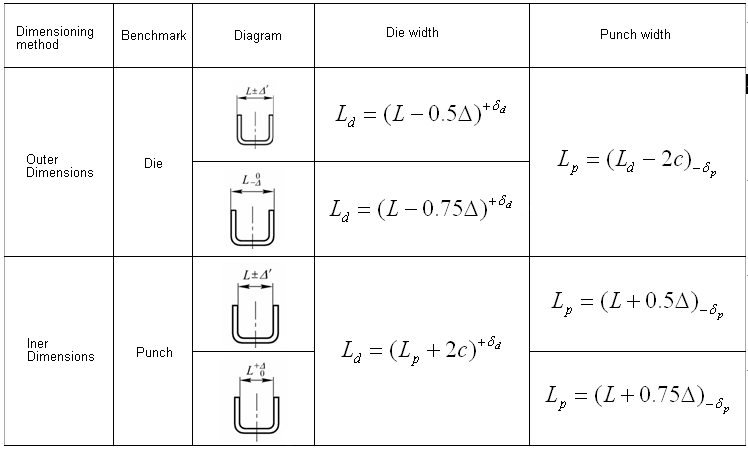2.Design of positioning parts

Since the blank fed into the bending die is a single blank, the positioning parts used in the bending die are positioning plates or pins.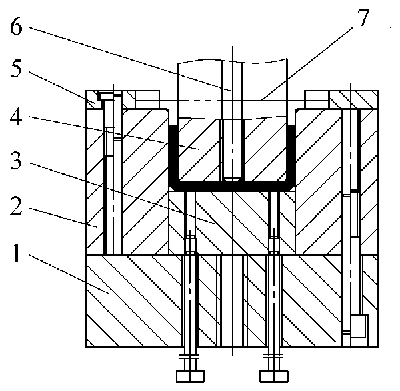3.Design of pressing, discharging and feeding parts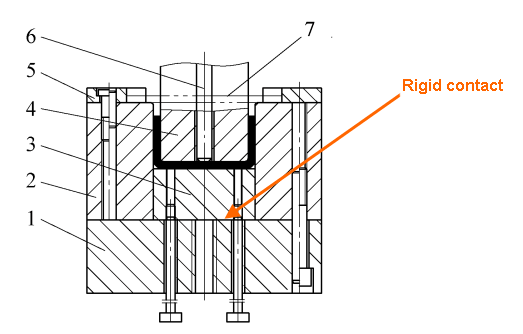4.Design of fixed parts

Including: die handle, upper die seat, lower die seat, guide post, guide sleeve, backing plate, fixing plate, screws, pins, etc., refer to the blanking die design.